# Olympiad Test: Alphabet Test -2

## 10 Questions MCQ Test Science Olympiad Class 7 | Olympiad Test: Alphabet Test -2

Description
Attempt Olympiad Test: Alphabet Test -2 | 10 questions in 20 minutes | Mock test for Class 7 preparation | Free important questions MCQ to study Science Olympiad Class 7 for Class 7 Exam | Download free PDF with solutions
QUESTION: 1

### Directions: In each of the following questions, find out which of the letter – series follows the given rule. Q. Number of letters skipped in between adjacent letters in the series is two.

Solution: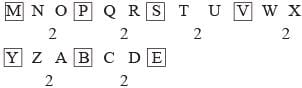QUESTION: 2

### Directions: In each of the following questions, find out which of the letter – series follows the given rule. Q. Number of letters skipped in between the adjacent letters in the series are consecutive even numbers.

Solution: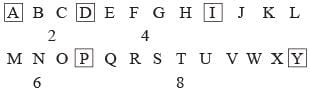2, 4, 6, 8 are consecutive even numbers.

QUESTION: 3

### Directions: In each of the following questions, find out which of the letter – series follows the given rule. Q. Number of letters skipped in between adjacent letters of the series starting from behind increase by one

Solution: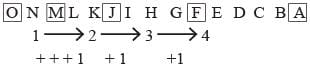QUESTION: 4

Directions: In each of the following questions, find out which of the letter – series follows the given rule.

Q. Number of letters skipped in between adjacent letters in the series decrease by two

Solution: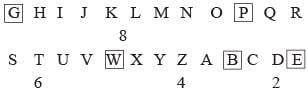QUESTION: 5

Directions: In each of the following questions, find out which of the letter – series follows the given rule.

Q. Number of letters skipped in between adjacent letters in the series doubles very lime.

Solution: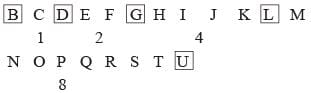QUESTION: 6

Directions: In each of the following questions, find out which of the letter – series follows the given rule.

In the following series, the number of letters skipped in between the adjacent letters are in ascending order, i.e., 1, 2, 2, 3 which one of the following letter groups does not obey this rule?

Solution: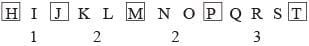QUESTION: 7

Directions: In each of the following questions, find out which of the letter – series follows the given rule.

Q. Number of letters skipped in between adjacent letters in the series is in the order of 2, 5, 7, 10.

Solution: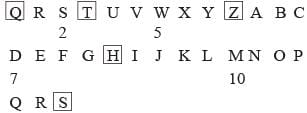QUESTION: 8

Directions: In each of the following questions, find out which of the letter – series follows the given rule.

The letters are not according to a general rule.

Solution: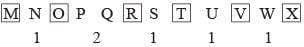QUESTION: 9

Directions: In each of the following questions, find out which of the letter – series follows the given rule.

Number of letters skipped in between adjacent letters in the series is odd.

Solution: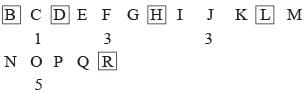QUESTION: 10

Directions: Study the following arrangement of the english alphabet and answer the questions given below:

Q. Which of the following pairs of letters has as many letters between them in the above arrangement as there are between them in the english alphabet?

Solution:

M and O have just one letter between them in the given series as well as in the english alphabet.Use Code STAYHOME200 and get INR 200 additional OFF Use Coupon Code